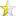Mar 28, 2015

# Fibonacii at its bestThis post will be about a problem statement that got in my college exams during my engineering. It was a question of an assignment. The question was as follows

Write the most efficient program to print Fibonacci series up to the value given during runtime and also store the values in a data structure to use it later..your code has to be very memory efficient and much better time complexity than normal programs. You can not use Dynamic Memory allocation!

Well to be precise there are a hell lot of solutions to find the answer. People do use it a lot of techniques to solve this question. But wait, there is a problem in this question. The problem is the choice of language. If you are interested to do this in the good old C, then the problem will occur at the point of storing the values.

You see in the question, it is mentioned that not only you have to print the series up to a certain value, but you need to save the data into a data structure. In C, we have only one rudimentary data structure which can save the series of data in a continuous memory locations: an array. But the problem with the array in C is that you can not declare a array of variable size. For example the line int a[size] would cause the program to crash. Hence you can not declare the size of the array during the runtime, which is actually the objective of the program.

The next solution is to use dynamic memory allocation like this

 ``1234567`` `````` int size; printf("Enter the length of the series :: "); scanf("%d", &size); int *memPtr = (int *)malloc( (size_t)( (int)sizeof(int) * size ) ); // insert the series.. free(memPtr);``````

But in the program it is explicitly mentioned that you can not use dynamic memory allocation, hence this option is not valid at all.

So the fact of the matter is that you can not design it in good old C..At least not that I know of. Hence I moved to C++ and after few tweaks & improvements I finally designed something which my professor liked & he finally accepted. Hence the objective of this article is to show my design & ask to fellow community members for a better solution if there were any possible.

I created an header file called fibo.h and the definition fibo.cpp , the main.cpp & of course the Makefile . Here are my each files

fibo.h
 ``12345678910111213`` `````` #ifndef _fibo_h_ #define _fibo_h_ #include class Fibonacii{ private: int size; std::vector data; public: Fibonacii(int); void create_series(void); void get_data(void); }; #endif // _fibo_h_ ``````

fibo.cpp
 ``1234567891011121314151617181920212223`` `````` #include "fibo.h" #include #include using namespace std; // This creates a Fibonacii series void Fibonacii::create_series(void){ data.push_back(0); data.push_back(1); for (int i = 2; i < size; ++i) { /* code */ data.push_back(data[i - 2] + data[i - 1]); } } // This is a constructor Fibonacii::Fibonacii(int s){ size = s; } // This method is used to print the series void Fibonacii::get_data(void){ for (long i: data) cout << i << endl; }``````

main.cpp
 ``12345678910111213141516`` `````` #include "fibo.h" #include #include #include using namespace std; int main(int argc, char *argv[]) { /* code */ if (argc == 2) { int value = stoul(argv, nullptr, 10); static Fibonacii Fibo(value); Fibo.create_series(); Fibo.get_data(); return 0; } }``````

Makefile
 ``123456789101112131415161718192021`` `````` MAIN = main HEADER_DEFINITIONS = fibo CC = g++-4.9 -std=c++11 COMPILE = -c EXE = \$(MAIN) OPTIMIZE = -Os SHELL = /bin/bash ARGS = 20 all: link @echo "Executing..........." @echo " > > > > > > OUTPUT < < < < < < " @\$(SHELL) -c './\$(EXE) \$(ARGS)' link: compile @echo -n "Linking............." @\$(SHELL) -c '\$(CC) -o \$(EXE) *.o' compile: \$(MAIN).cpp \$(HEADER_DEFINITIONS).cpp @echo -n "Compiling........." @\$(SHELL) -c '\$(CC) \$(OPTIMIZE) \$(COMPILE) \$^' clean: @echo "Cleaning............" @\$(SHELL) -c 'rm -f *~ *.o \$(EXE)'``````

[NOTE: if you don't have g++4.9 version, use only g++. But don't forget to put -std=c++11]

[NOTE: vector is type of data structure that as much as I know is implemented using a class template and dynamic memory allocation. Hence this program is still using dynamic memory allocation indirectly]

[NOTE: if you need to change the length of the series, the edit the value of ARGS = 20 into any value you like]

To run the program, just move to the directory in your terminal and type make all